The development of solar energy is constantly evolving. This web also.

Here we offer our new products and the latest updated articles.

## Imaginary Earth lines, meridians and parallels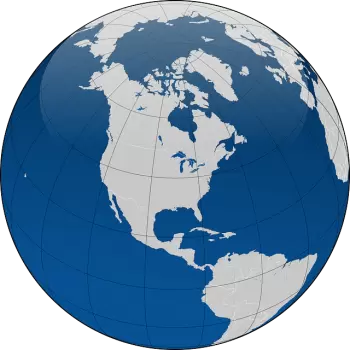The imaginary lines of the Earth are lines drawn on the planisphere map in which the continents and the oceans that go from this to west and from north to south are represented. These lines are called meridians and parallels.

The meridians and parallels form a geographical grid thanks to which it is possible to orient oneself. We can identify any point on Earth if we know its geographic coordinates: latitude and longitude.

In particular, assuming that the Earth is in the shape of a sphere, the imaginary lines form a circle.

What are the Earth's meridians?

Meridians are imaginary circles that cross the earth's surface through the North Pole and the South Pole.

Last review: September 24, 2021

## What is the distance from the Earth to the Sun?The distance that separates the Sun from the Earth is an average of 150 million km. This distance is used as a reference to measure distances in the solar system: the average distance from the Sun to the Earth corresponds to 1 AU.

The Earth, like the other celestial bodies in the solar system, orbits around the Sun, but it does not follow a circular orbit but rather describes an elliptical path, the Sun being one of the foci of the ellipse.

Earth is the third planet in order of distance from the Sun; both by mass and diameter it is the largest among the terrestrial planets (that is, among the planets composed of rock and metals) in the solar system.

The Sun is the king…

Last review: September 23, 2021

## How does a forced circulation solar system work?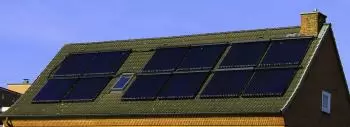A forced circulation solar system is a solar thermal installation in which the water circulates within the circuit driven by a pump.

Unlike solar installations with a thermosyphon, forced circulation water heating systems do not move the hot water to the highest point of the closed circuit. Instead, the pump makes water go down from the solar collectors to the accumulation tank.

On many occasions, installing solar thermal energy equipment to obtain sanitary hot water by thermosyphon is not feasible since the solar collectors often are above the accumulator.

This renewable energy system is a must-have in underfloor solar water heating.

In these types of solar thermal…

Last review: September 21, 2021

## What is a thermal power plant? Operation and types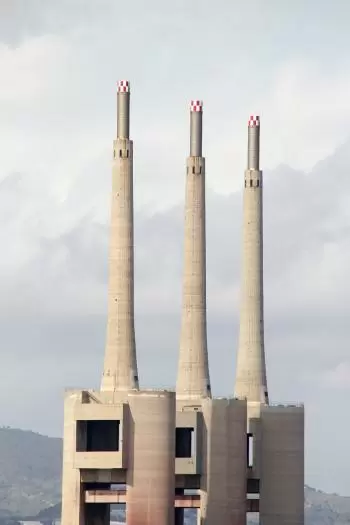A thermal power plant is an electric plant that converts thermal energy into electrical energy. The difference between one type of plant and another is the way the heat is obtained.

The heat sources of a thermal power plant are:

• Fossil fuels (coal, diesel and natural gas)
• Nuclear reactions of uranium atoms.
• Biomass.
• Solar energy.

The first two are non-renewable energy sources. On the other hand, biomass and solar energy are considered renewable energy even though the former emits greenhouse gases and solar thermal power plants do not.

How does a conventional…

Last review: September 18, 2021

## Balance of system (BOS) in a photovoltaic solar facility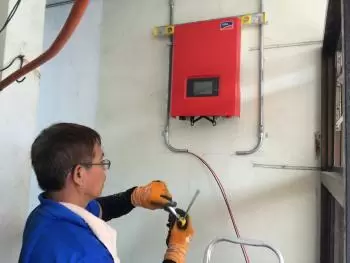The balance of system (also known by the acronym BOS) includes all the photovoltaic system components except for the photovoltaic panels.

We can think of a complete photovoltaic energy system of three subsystems when we speak about solar energy.

• On the power generation side, a subsystem of photovoltaic devices (solar cells, PV modules, arrays) converts sunlight into direct current (DC) electricity.

• On the energy use side, the subsystem consists mainly of charging, which is the application of photovoltaic electricity.

• Between these two, we need a third subsystem that allows the electricity generated by the photovoltaic modules to…

Last review: September 17, 2021

## Ampère's law, formula and example of applications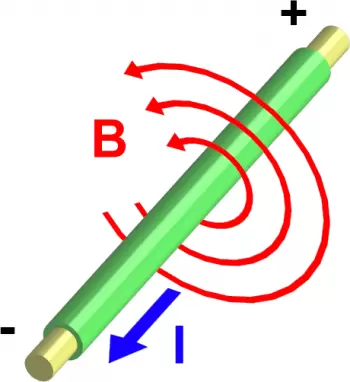Ampère's law is one of the fundamental laws of classical electrodynamics. This law was formulated by Andre Marie Ampère in 1826.

Ampère's law states:

• The circulation of the magnetic field of constant currents along any closed circuit is proportional to the sum of the forces of the currents that cross the surface of the circuit.

• If direct current is used, the magnetic field is continuous.

• If alternating current is used, the magnetic field is alternating.

Ampère's law formula

Ampère's law can be represented by the following equation:

Last review: September 15, 2021

## State Joule’s law of heating. What is Joule's effect?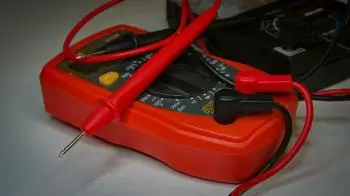The Joule effect, also called Joule's law, is the thermal manifestation of electrical resistance. It states that the heat generated due to the current that flows along a conductor is proportional to the electrical resistance, the amperage, and the time.

Joule’s law also states that the internal energy of an ideal gas is solely a function of its temperature.

If electricity circulates in an electrical conductor, a part of the kinetic energy of the electrons is transformed into heat, raising the temperature of the conductor.

The heat is generated due to the collision that electrons experience…

Last review: September 14, 2021

## What is Ohm's Law? Definition, formula and exampleOhm's law is a mathematical formula that describes the correlation of electrical parameters (resistance, current, voltage) with which they vary.

The name of the law is due to the German physicist Georg Ohm.

Definition of Ohm's law

Ohm's law is a law in electricity that states that: "the force of a direct current is directly proportional to the potential difference and inversely proportional to the resistance of the circuit."

Ohm's law formula

In mathematical terms, Ohm's law is applied by the equation:

Last review: September 13, 2021

## Laws of electricity, concepts and descriptionsElectricity is the movement of electrical charges that circulate through a conductor. This movement is carried out according to certain physical properties. These properties are collected in a series of laws and theorems that scientists have developed throughout history.

The most important laws and theorems related to electrical energy are:

Coulomb's law

Coulomb's law states that the electric force of two charged objects is inversely proportional to the square of the distance between them. Coulomb's law also says that this force is directly proportional to the product of the charges.

This law was first introduced in 1785 by the physicist Charles-Augustin…

Last review: September 12, 2021

## Which device provides electrical energy to run an electric circuit?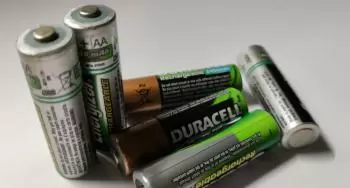The generator of an electric circuit is a device capable of creating a difference between the negative and positive terminals. An electrical generator is a device that converts a type of energy into electrical energy so that this energy can be used in an external electrical circuit.

An electrical circuit needs a source of energy, a current generator that drives electrons.

Concerning the question about which device provides electrical energy to run an electric circuit, the response is that there are several. Electrical generators in electrical circuits can use different sources of energy to convert it into electrical energy. The most common energy sources are:

• Chemical…

Last review: September 11, 2021